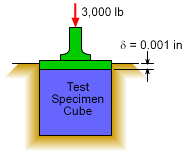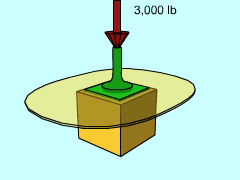Ch 1. Stress and Strain Multimedia Engineering Mechanics NormalStress Shear andBearing Stress NormalStrain Hooke'sLaw ThermalEffects IndeterminateStructures
 Chapter 1. Stress/Strain 2. Torsion 3. Beam Shr/Moment 4. Beam Stresses 5. Beam Deflections 6. Beam-Advanced 7. Stress Analysis 8. Strain Analysis 9. Columns Appendix Basic Math Units Basic Equations Sections Material Properties Structural Shapes Beam Equations Search eBooks Dynamics Fluids Math Mechanics Statics Thermodynamics Author(s): Kurt Gramoll ©Kurt GramollMECHANICS - CASE STUDY IntroductionCross Section of Test SystemSpecimen Testing Set Up Susan Placer, a new engineer at AAA Materials Testing Lab, is asked to find the Poisson's ratio for a new epoxy adhesive. The test method used at the lab compresses a 1 in cube specimen under compression. The test frame consists of a 1 in cube hole to place the sample. Then a load is place on the top surface (see figure). The total deflection of the top surface is monitored. The test sample is a 1 in cube which is placed into a perfectly fitting frame. The frame is substantially more stiff than the sample and will not deflect during the test. What is known: Young modulus, E, is 2,000 ksi. The frame is much stiffer than the specimen. The cube dimension is 1 in on each side. At 3,000 lbs, the cube deflects 0.001 in. The sides are frictionless. There are no gaps when sample is in the frame. Question What is the Poisson's ratio of the new epoxy adhesive? Also, what stresses are generated on the sides of the test frame? Approach Since the frame is much stiffer than the specimen, the side deflections can be assumed equal to zero. Stress in the x and y directions (horizontal sides of box) are not zero. Use the three dimensional equations to set up the problem. First, determine Poisson's ratio. Second, determine the stress on each side using the Poisson's ratio.

Practice Homework and Test problems now available in the 'Eng Mechanics' mobile app
Includes over 400 problems with complete detailed solutions.
Available now at the Google Play Store and Apple App Store.